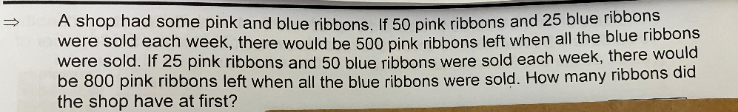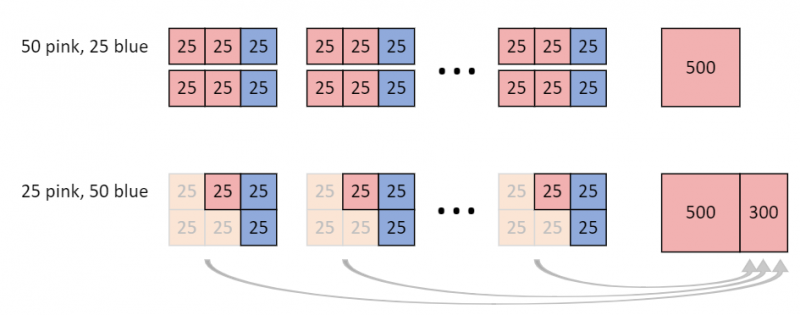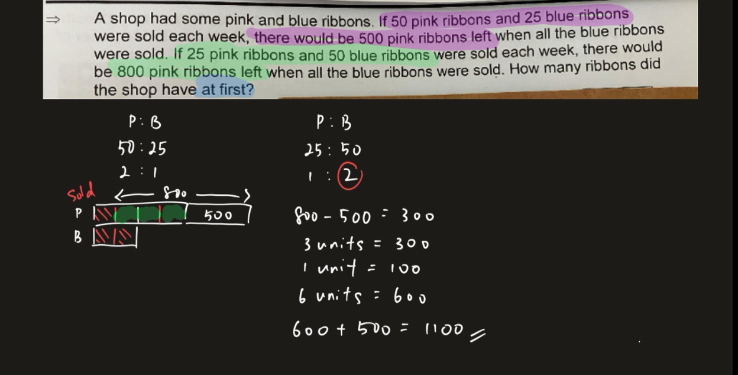# QuestionThank you.

```The extra 300 ribbons comes from the groups of 75 ribbons (greyed out boxes).
There are 300/75 = 4 groups (of 25 pink, 50 blue in 2nd row).
So total is 4 x (25 pink + 50 blue) + 800 pink = 1100 ribbons.

No algebra! :-P

CHECK:
4 x 50 blue = 200 blue. Top row has 200/25 = 8 groups (of 50 pink, 25 blue).
Total in top row: 8 x (50 pink + 25 blue) + 500 = 1100 ribbons.

```
0 Replies 4 Likes ✔Accepted Answer

For the blue ribbons sold in case 1 and case 2 (the blue ribbons are equal in both cases),

So  25(number of weeks sold) = 50 (number of weeks sold)

This means the number of weeks in case 1 is twice the number of weeks in case 2.

So let the number of weeks in case 2 be u, then number of weeks in case 1 = 2u

Pink ribbons in case 1 = pink ribbons in case 2 :

50(2u)+500 = 25u + 800

75u = 300 => u=4

So pink ribbons = 25(4) + 800 = 900

blue ribbons = 50(4) = 200

Total ribbons = 900+200 = 1100

0 Replies 4 LikesVideo Solution can be viewed Here

0 Replies 2 Likes